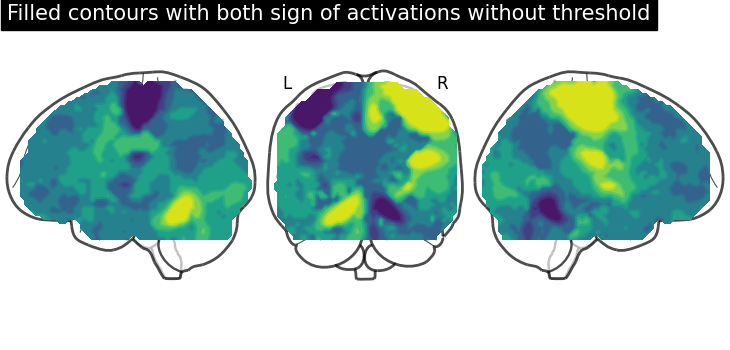# Glass brain plotting in nilearn (all options)#

The first part of this example goes through different options of the `plot_glass_brain` function (including plotting negative values).

The second part goes through same options but selected of the same glass brain function but plotting is seen with contours.

See Plotting brain images for more plotting functionalities and Section 4.3 for more details about display objects in Nilearn.

Also, see `nilearn.datasets.fetch_neurovault_motor_task` for details about the plotting data and associated meta-data.

We will use a motor activation contrast map distributed with Nilearn.

```from nilearn import datasets

# stat_img is just the name of the image file
stat_img
```
```'/home/yasmin/nilearn/nilearn/nilearn/datasets/data/image_10426.nii.gz'
```

## Demo glass brain plotting#

By default, `plot_glass_brain` uses a display mode called ‘ortho’ which results in three projections. It is equivalent to specify `display_mode='ortho'` in `plot_glass_brain`. Note that depending on the value of `display_mode`, different display objects are returned. Here, a `OrthoProjector` is returned.

```from nilearn import plotting
from nilearn.plotting import plot_glass_brain

# Whole brain sagittal cuts and map is thresholded at 3
plot_glass_brain(stat_img, threshold=3)
```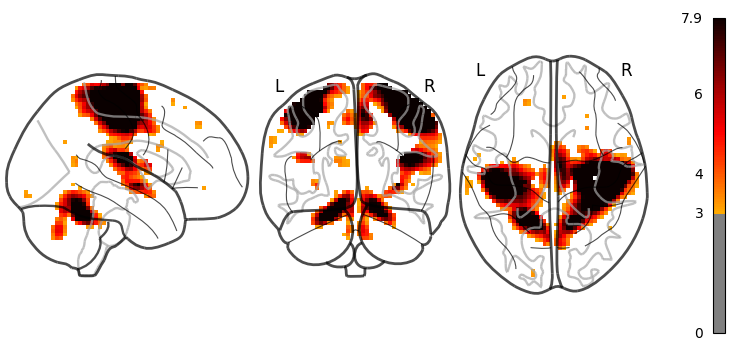```<nilearn.plotting.displays._projectors.OrthoProjector object at 0x7fb57c89db10>
```

The same figure, with a colorbar, can be produced by setting `colorbar=True`.

```plot_glass_brain(stat_img, threshold=3, colorbar=True)
```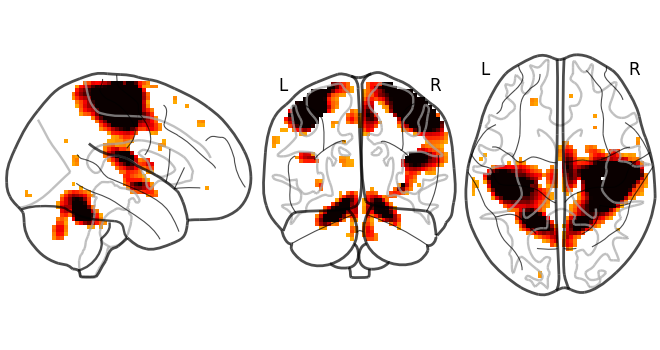```<nilearn.plotting.displays._projectors.OrthoProjector object at 0x7fb585ad16f0>
```

Here, we show how to set a black background, and we only view sagittal and axial projections by setting `display_mode='xz'`, which returns a `XZProjector`.

```plot_glass_brain(
stat_img, title='plot_glass_brain', black_bg=True,
display_mode='xz', threshold=3
)
```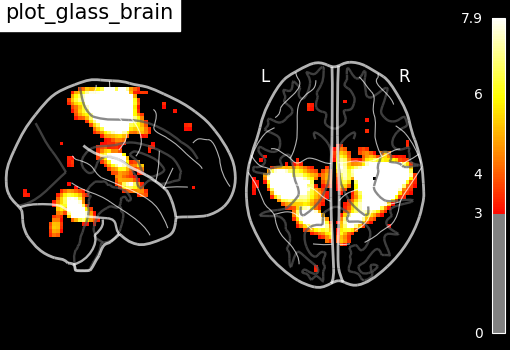```<nilearn.plotting.displays._projectors.XZProjector object at 0x7fb58debca60>
```

We can also plot the sign of the activation by setting `plot_abs=False`. Additionally, we only visualize coronal and axial projections by setting `display_mode='yz'` which returns a `YZProjector`.

```plot_glass_brain(
stat_img, threshold=0, colorbar=True, plot_abs=False, display_mode='yz'
)
```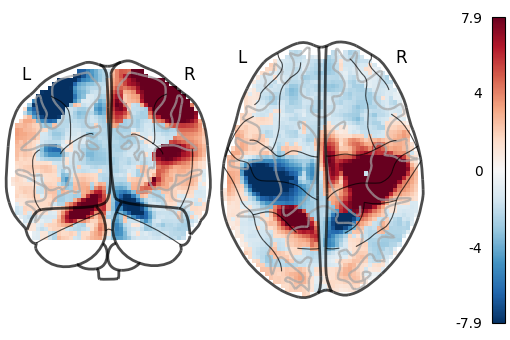```<nilearn.plotting.displays._projectors.YZProjector object at 0x7fb590762d10>
```

Setting `plot_abs=True` and `display_mode='yx'` (returns a `YXProjector`).

```plot_glass_brain(
stat_img, threshold=3, colorbar=True, plot_abs=False, display_mode='yx'
)
```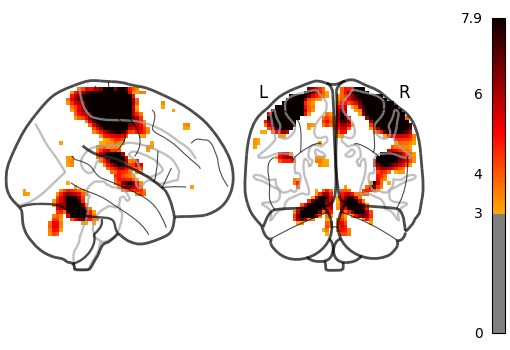```<nilearn.plotting.displays._projectors.YXProjector object at 0x7fb57d25bfd0>
```

## Different projections for the left and right hemispheres#

In the previous section we saw a few projection modes, which are controlled by setting the argument `display_mode` of `plot_glass_brain`. In this section, we will show some additional possibilities. For example, setting `display_mode='lzr'` enables an hemispheric sagittal view. The display object returned is then a `LZRProjector`.

```plot_glass_brain(
stat_img, title='plot_glass_brain with display_mode="lzr"',
black_bg=True, display_mode='lzr', threshold=3
)
```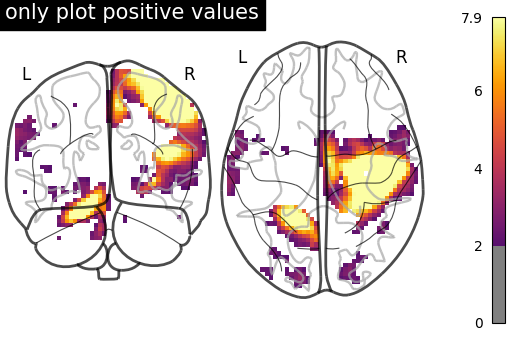```<nilearn.plotting.displays._projectors.LZRProjector object at 0x7fb5906bc340>
```

`display_mode='lyrz'` returns a `LYRZProjector` object.

```plot_glass_brain(
stat_img, threshold=0, colorbar=True,
title='plot_glass_brain with display_mode="lyrz"',
plot_abs=False, display_mode='lyrz'
)
```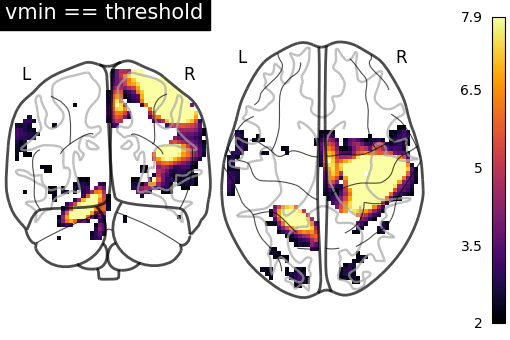```<nilearn.plotting.displays._projectors.LYRZProjector object at 0x7fb5acc04f10>
```

If you are only interested in single projections, you can set `display_mode` to ‘x’ (returns a `XProjector`), ‘y’ (returns a `YProjector`), ‘z’ (returns a `ZProjector`), ‘l’ (returns a `LProjector`), or ‘r’ (returns a `RProjector`).

```plot_glass_brain(
stat_img, threshold=0, colorbar=True, title='display_mode="x"',
plot_abs=False, display_mode='x'
)
```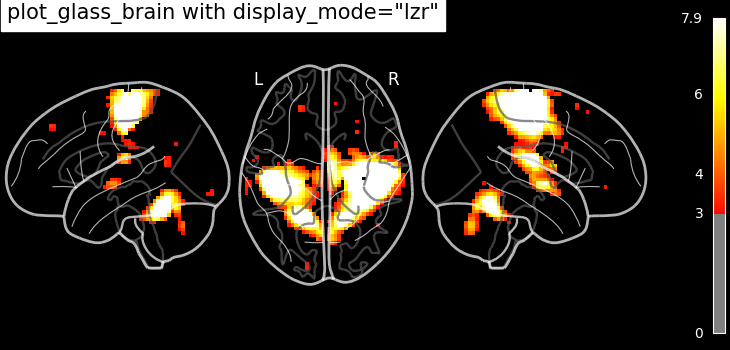```<nilearn.plotting.displays._projectors.XProjector object at 0x7fb596a26ec0>
```

## Demo glass brain plotting with contours and with fillings#

The display objects returned by `plot_glass_brain` all inherit from the `OrthoProjector` and enable further customisation of the figures.

In this example, we focus on using methods `add_contours` and `title`. First, we save the display object (here a `LZRYProjector`) into a variable named `display`. Note that we set the first argument to `None` since we want an empty glass brain to plot the statistical maps with `add_contours`.

```display = plot_glass_brain(None, display_mode='lzry')
# Here, we project statistical maps
display.title('"stat_img" on glass brain without threshold')
```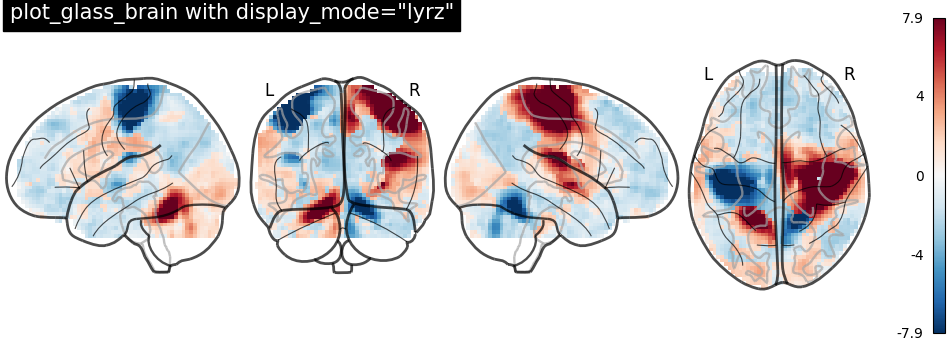We can fill the contours by setting `filled=True`. Note that we are not specifying levels here

```display = plot_glass_brain(None, display_mode='lzry')
# Here, we project statistical maps with filled=True
display.title('Same map but with fillings in the contours')
```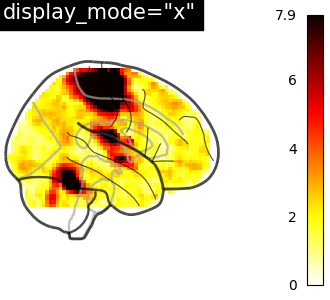Here, we input a specific level (cut-off) in the statistical map. In other words, we are thresholding our statistical map.

We set the threshold using a parameter of method `add_contours` called `levels` which value is given as a list and we choose the color to be red.

```display = plot_glass_brain(None, display_mode='lzry')
display.title('"stat_img" on glass brain with threshold')
```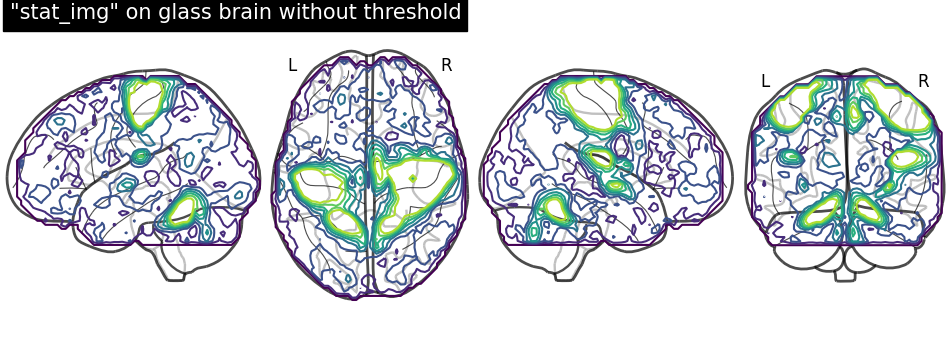Plotting with same demonstration but fill the contours (by setting `filled=True`).

```display = plot_glass_brain(None, display_mode='lzry')
display.title('Same demonstration but using fillings inside contours')
```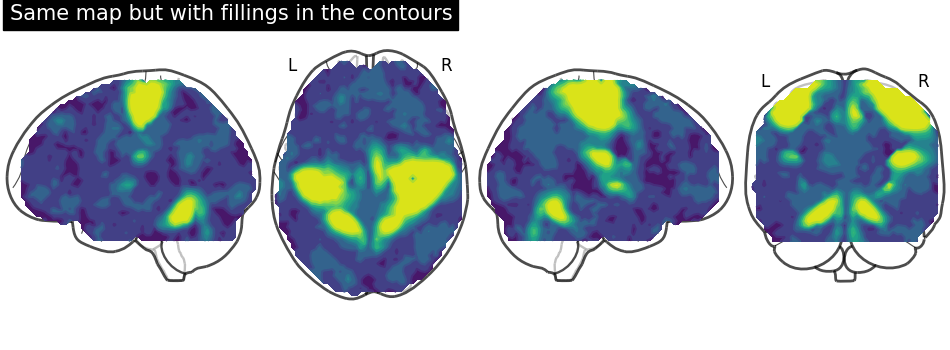Plotting with black background, `black_bg` should be set to `True` through `plot_glass_brain`.

```# We can set black background using black_bg=True
display = plot_glass_brain(None, black_bg=True)
display.title('"stat_img" on glass brain with black background')
```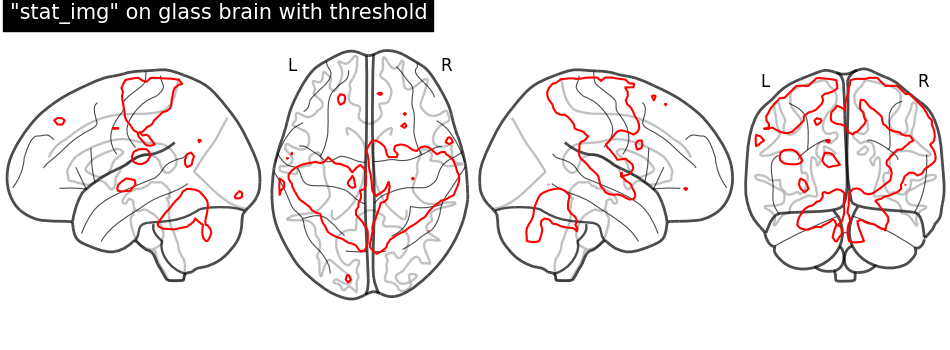Black background plotting with filled in contours.

```display = plot_glass_brain(None, black_bg=True)
display.title('Glass brain with black background and filled in contours')
```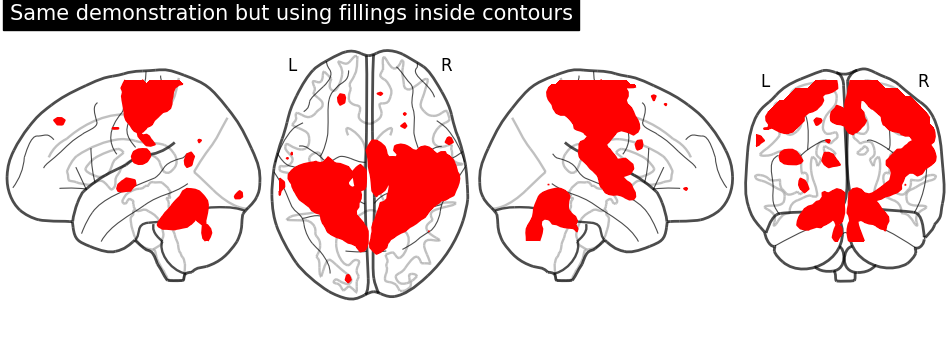## Display contour projections in both hemispheres#

The key argument to vary here is `display_mode` for hemispheric plotting. Here, we set `display_mode='lr'` for both hemispheric plots. Note that a `LRProjector` is returned.

```display = plot_glass_brain(None, display_mode='lr')
display.title('"stat_img" on glass brain only "l" "r" hemispheres')
```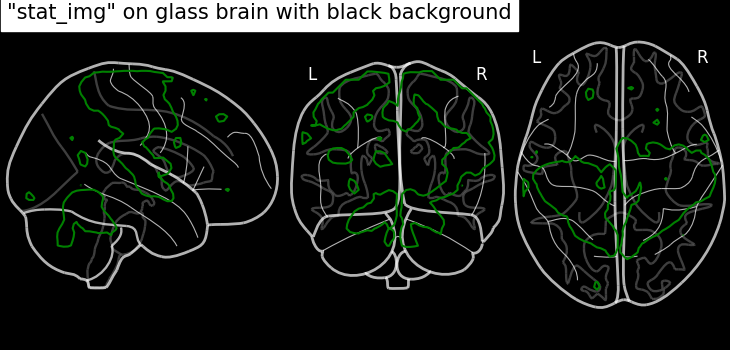Filled contours in both hemispheric plotting, by adding `filled=True`.

```display = plot_glass_brain(None, display_mode='lr')
display.title('Filled contours on glass brain only "l" "r" hemispheres')
```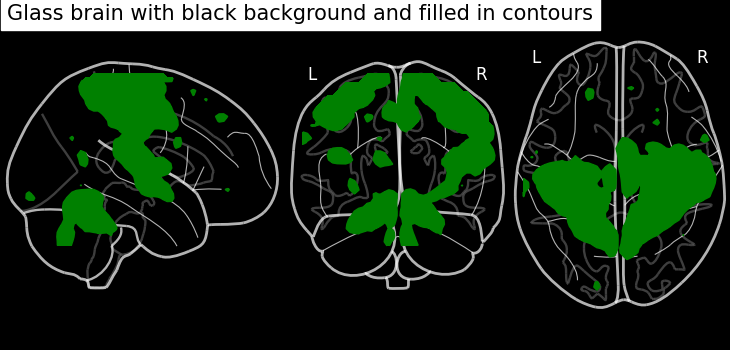With positive and negative signs of activations with `plot_abs` in `plot_glass_brain`.

By default parameter `plot_abs` is `True` and sign of activations can be displayed by changing `plot_abs` to `False`. Note that we also specify `display_mode='lyr'` which returns a `LYRProjector` display object.

```display = plot_glass_brain(None, plot_abs=False, display_mode='lyr')
display.title("Contours with both sign of activations without threshold")
```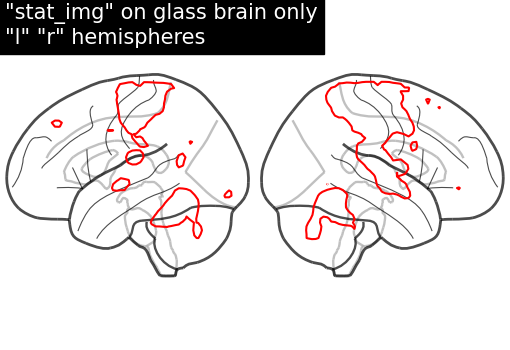Now, adding `filled=True` to get positive and negative sign activations with fillings in the contours.

```display = plot_glass_brain(None, plot_abs=False, display_mode='lyr')
display.title(
"Filled contours with both sign of activations without threshold"
)
```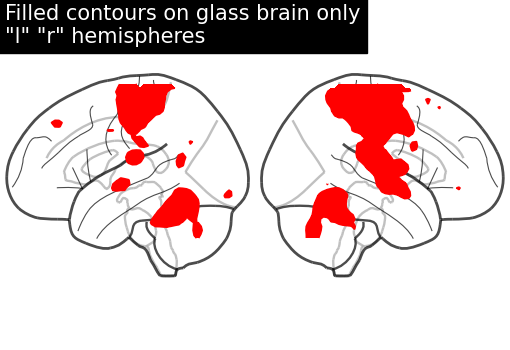Displaying both signs (positive and negative) of activations with threshold meaning thresholding by adding an argument `levels` in method `add_contours`.

We give two values through the argument `levels` which corresponds to the thresholds of the contour we want to draw: One is positive and the other one is negative. We give a list of `colors` as argument to associate a different color to each contour. Additionally, we also choose to plot contours with thick line widths. For `linewidths`, one value would be enough so that same value is used for both contours.

```import numpy as np

display = plot_glass_brain(None, plot_abs=False, display_mode='lzry')
stat_img, levels=[-2.8, 3.], colors=['b', 'r'], linewidths=4.
)
display.title('Contours with sign of activations with threshold')
```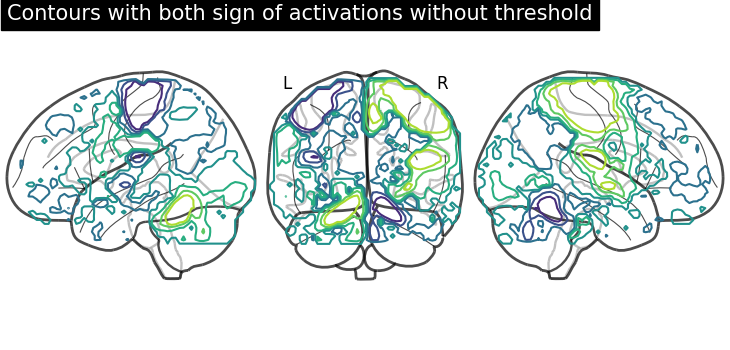Same display demonstration as above but adding `filled=True` to get fillings inside the contours.

Unlike in previous plot, here we specify each sign at a time. We call negative values display first followed by positive values display.

First, we fetch our display object with same parameters used as above. Then, we plot negative sign of activation with levels given as negative activation value in a list. Upper bound should be kept to -infinity. Next, using the same display object, we plot positive sign of activation.

```display = plot_glass_brain(None, plot_abs=False, display_mode='lzry')
stat_img, filled=True, levels=[-np.inf, -2.8], colors='b'
)